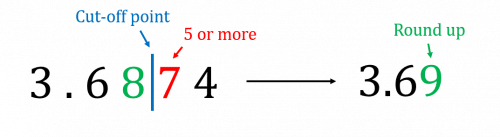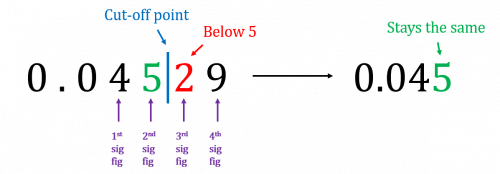# Rounding Numbers

GCSEKS3Level 1-3AQACambridge iGCSEEdexcelEdexcel iGCSEOCRWJEC

## Rounding Numbers

Rounding is a process of simplifying a number without changing the value of it too much. We do this by taking a number and working out which of the “simpler” numbers it is closest to.

If you round numbers too early, i.e. during a calculation, then the answer you get at the end may be incorrect, so  only round at the end of an answer, and not during a calculation, unless the question specifically asks you to.

Make sure you are happy with the following topics before continuing.

Level 1-3GCSEKS3AQAEdexcelOCRWJECCambridge iGCSEEdexcel iGCSE

## Skill 1: Rounding – Decimal Places

One way we may be asked to round a number is to a given number of decimal places (d.p.).

Example: Round $3.6874$ to $2$ decimal places.

Step 1: Determine the cut-off point.

Here, this is the $2$nd decimal place. The cut-off point is after the $8$ and before the $7$.

Step 2: Look at the first digit after the cut-off point. Here it is the $7$.

If it is below $5$, then we keep the cut-off digit (i.e. the number before the cut-off point) the same.

If it is $5$ or more, then we round up the cut-off digit.Here, $7$ is more than $5$, so we round the cut-off digit, $8$, up to $9$.

Step 3: All the digits after the cut-off point we ignore. So,

$3.6874$ rounded to $2$ decimal places is $3.69$

Level 1-3GCSEKS3AQAEdexcelOCRWJECCambridge iGCSEEdexcel iGCSE

## Skill 2: Rounding – Significant Figures

Another way we may be asked to round a number is to a given number of significant figures (sf). The $1$st significant figure of a number is the first digit that isn’t a $0$. The next significant figures immediately follows, and so on…..

Example: Round $0.04529$ to $2$ significant figures.

Step 1: Determine the cut-off point.

Here this is after the $2$nd significant figure. The $1$st significant figure is $4$ as the first $2$ digits are zeros.

The $2$nd significant figure is the $5$.

The cut-off point is between the $5$ and the $2$.

Step 2: Look at the first digit after the cut-off point. Here it is the $2$.

If it is below $5$, then we keep the cut-off digit the same.

If it is $5$ or more, then we round up the cut-off digit.Here, $2$ is less than $5$, so we keep the cut-off digit, $5$, the same.

Step 3: All the digits after the cut-off point we ignore. So,

$0.04529$ rounded to $2$ significant figures is $0.045$

Level 1-3GCSEKS3AQAEdexcelOCRWJECCambridge iGCSEEdexcel iGCSE

## Skill 3: Rounding – Nearest Whole Number, 10, 100 etc.

Finally, we may be asked to round a number to the nearest whole number, $10$, $100$ etc.

This is easier than for decimal places and significant figures. We use the same method, but if it asks to round to the nearest $10$ for example, then our cut-off digit will be the digit in the tens column.

Example: Round $112$ to the nearest ten

Step 1: Identify the tens digit, here it will be the second $1$

$1\overset{\downarrow}{1}2$

Step 2: Look at the digit after the tens column. Here it is $2$

If it is below $5$, then we keep the cut-off digit the same.

If it is $5$ or more, then we round up the cut-off digit.

Here, $2$ is less than $5$ so we do not round up.

Step 3: All the digits after the cut-off digit we turn into $0$‘s.

This gives the final answer to be:

$110$

Level 1-3GCSEKS3AQAEdexcelOCRWJECCambridge iGCSEEdexcel iGCSE

## Skill 4: Rounding – Carrying the 1

For each of the $3$ ways of rounding, if we are required to round up a $9$, then we round the $9$ to a $0$ and increase the digit before it by $1$ (carry the $1$ to the left).

Example: Round $4.398$ to $2$ decimal places.

The $2$nd digit after the decimal point, the $9$, is our cut-off digit. The digit after it is an $8$, so we must round up the cut-off digit.

The problem is, increasing a $9$ by $1$ makes $10$, so what do we do? The cut-off digit becomes zero, and the digit before it gets increased by $1$. So, in this case, the $9$ goes to zero and the $3$ before it becomes $4$.

Then, ignoring all the digits after the cut-off point, we get

$4.398$ rounded to $2$ decimal places is $4.40$

Level 1-3GCSEKS3AQAEdexcelOCRWJECCambridge iGCSEEdexcel iGCSE
Level 1-3GCSEKS3AQAEdexcelOCRWJECCambridge iGCSEEdexcel iGCSE

## Example 1: Rounding to the Nearest 10

Round $235$ to the nearest ten.

[1 mark]

The first digit, $2$, is in hundreds, but the second digit, $3$, is in tens, so that will be our cut-off digit.

Now we look to the digit after this: it is a $5$, which means that we round up, i.e. the cut-off digit gets increased by $1$, so in this case the $3$ will become a $4$.

Finally, turning all the digits after the cut-off point to $0$ ‘s, we get that

$235$ rounded to the nearest $10$ is $240$

Level 1-3GCSEKS3AQAEdexcelOCRWJECCambridge iGCSEEdexcel iGCSE

## Example 2: Rounding Decimals

Round $13.746$ to $2$ decimal places.

[1 marks]

If we’re rounding to $2$ decimal places, then the cut-off digit is before the $2$nd decimal place, i.e. the $2$nd number after the decimal point. In this case, that is the $4$.

The digit after the $4$ is a $6$, which means that we round up and increase the cut-off digit by $1$ so the $4$ becomes a $5$, giving us

$13.746$ rounded to $2$ decimal places is $13.75$

Level 1-3GCSEKS3AQAEdexcelOCRWJECCambridge iGCSEEdexcel iGCSE

## Example 3: Rounding Significant Figures

Round $8,529$ to $2$ significant figures.

[1 mark]

The $1$st significant figure is $8$, the $2$nd is $5$.

The $5$ is our cut-off digit.

The digit after the $5$ is a $2$. This is less than $5$, so we round down and the cut-off digit stays the same.

Then, turning all the digits after the cut-off digit into $0$‘s, we get

$8,529$ rounded to $2$ significant figures is $8,500$

Level 1-3GCSEKS3AQAEdexcelOCRWJECCambridge iGCSEEdexcel iGCSE

## Example 4: Rounding Significant Figures

Round $0.00589$ to $2$ significant figures.

[1 mark]

The first non-zero term is $5$, so this is our $\, 1$st significant figure. $8$, is our $2$nd significant figure and thus is our cut-off digit.

The digit after the $8$ is a $9$, which is bigger than $5$, and so we round up, increasing the cut-off digit by $1$, making the $8$ into a $9$.

Then, ignoring all the digits after the cut-off point, we get

$0.00589$ rounded to $2$ significant figures is $0.0059$

Level 1-3GCSEKS3AQAEdexcelOCRWJECCambridge iGCSEEdexcel iGCSE

## Rounding Numbers Example Questions

Here, we are rounding to the nearest thousand so it is the $3$rd digit we are interested in,

$560,180$

To round up or down we have to consider the value of the digit to its immediate right which in this case is $1$.

As $1$ is less than $5$, we round down and so the zero stays the same and we make the rest of the digits zero,

$560,180$ rounded to the nearest thousand is $560,000$Gold Standard Education

Here, we are rounding to $1$ decimal place so it is the $1$st digit after the decimal point we are interested in,

$97.96$

To round up or down we have to consider the value of the digit to its immediate right which in this case is $6$.

As $6$ is greater than $5$, we round up. However, as we are rounding up $9$ to $10$, we have to carry the $1$ over to the units column, so we get,

$97.96$ rounded to $1$ decimal place is $98.0$Gold Standard Education

Here, we are rounding to $3$ significant figures so it is the first non-zero digit and the two digits that follow it that we are interested in,

$0.02345$

The $1$st significant figure in this case is $2$ and moving two places along we see that the $4$ is the $3$rd significant figure. To round up or down we have to consider the value of the digit to its immediate right which in this case is $5$, so we round up,

$0.02345$ rounded to $3$ significant figures is $0.0235$Gold Standard Education

Here, we are rounding to $3$ significant figures so it is the first non-zero digit and the two digits that follow it that we are interested in,

$1.0093$

The $1$st significant figure in this case is $1$ and moving two places along we see that the $0$ is the $3$rd significant figure. To round up or down we have to consider the value of the digit to its immediate right which in this case is $9$, so we round up,

$1.0093$ rounded to $3$ significant figures is $1.01$Gold Standard Education

Here, we are rounding to $2$ decimal places so it is the $2$nd digit after the decimal point we are interested in,

$55.099$

To round up or down we have to consider the value of the digit to its immediate right which in this case is $9$. As $9$ is greater than $5$, we round up. However, as we are rounding up $9$ to $10$, we have to carry the $1$ over to the units column, so we get,

$55.099$ rounded to $2$ decimal places is $55.10$Gold Standard Education

## Rounding Numbers Worksheet and Example Questions

### (NEW) Rounding Numbers - Exam Style Questions

Level 1-3GCSENewOfficial MME

## Rounding Numbers Drill Questions

### Rounding - Drill Questions

Level 1-3GCSEGold Standard Education

£19.99 /month. Cancel anytime

Level 1-3GCSEKS3

Level 1-3GCSEKS3